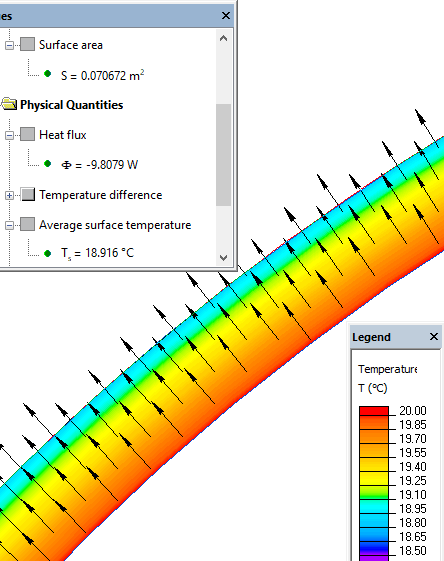QuickField

A new approach to field modelling
 Language: Global English Deutch Espanol Francais Italiano Danmark Ceske Chinese Pycckuü

Main >> Applications >> Sample problems >> Natural convection from the spherical surface

# Natural convection from the spherical surface

Steel sphere divides two air environments with different temperatures.

Problem type:
Axisymmetric problem of steady-state heat transfer.

Geometry:Sphere radius R = 75 mm, thickness of the sphere's wall d = 3 mm.

Given:
temperature of the inner surface of the sphere T0 = 20 °C,
temperature of the air outside the sphere T = -10 °C
thermal conductivity of the plate λplate = 0.4 W/(m·K)

Calculate the temperature of the outer surface of the sphere and the heat flux passing through the surface.

Solution:
The heat flux flows from hot to cold areas (in our case from inner surface of the sphere, then through the sphere toward air outside). Heat flux in the sphere is regulated by the sphere thermal conductivity. The heat exchange between air and the sphere is regulated by natural convection. We estimate that the temperatures of the sphere wall is equal to the inner surface temperature:
Tsphere = T0 = 20 °C.
This value is used for the convection coefficient calculation*:
αsurf = 4.8 W/(m2·K).

Result:
Temperature of the external surface of the sphere: Tsurf = 19 °C.
Heat flux: F = 10 W (sphere surface area is 0.070 m2).*Remark: the formulas of the similarity theory gives the average value of the convection coeffitient. This approach is very approximate by its nature, so there is no reason to make iterations to calculate the accurate Nusselt number related to actual surfaces temperatures.

• View simulation report in PDF
• Download simulation files (files may be viewed using any QuickField Edition).